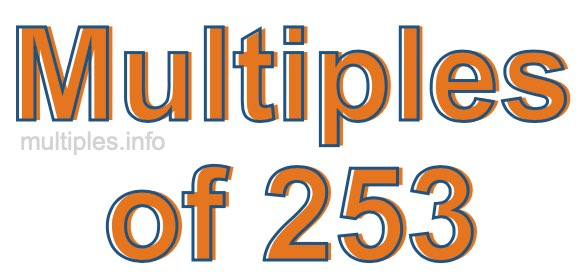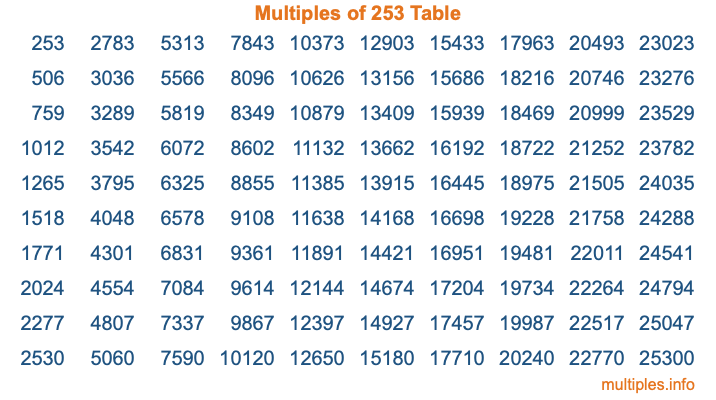Multiples of 253Welcome to the Multiples of 253 page. Here we will first teach you everything you will ever need to know about the multiples of 253, and then give you a study guide summary of everything we taught you to make sure you remember it all. Use this page to look up facts and learn information about the multiples of 253. This page will make you a multiples of two hundred fifty-three expert!

Definition of Multiples of 253
Multiples of 253 are all the numbers that when divided by 253 equal an integer. Each of the multiples of 253 are called a multiple. A multiple of 253 is created by multiplying 253 by an integer.

Therefore, to create a list of multiples of 253, you start with 1 multiplied by 253, then 2 multiplied by 253, then 3 multiplied by 253, and so on for as long as you want. Thus, the list of the first five multiples of 253 is 253, 506, 759, 1012, and 1265. To see a larger list of multiples of 253, see the printable image of Multiples of 253 further down on this page. We also have a category where you can choose any nth multiple of 253.

Multiples of 253 Checker
The Multiples of 253 Checker below checks to see if any number of your choice is a multiple of 253. In other words, it checks to see if there is any number (integer) that when multiplied by 253 will equal your number. To do that, we divide your number by 253. If the the quotient is an integer, then your number is a multiple of 253.

Is  a multiple of 253?

Least Common Multiple of 253 and ...
A Least Common Multiple (LCM) is the lowest multiple that two or more numbers have in common. This is also called the smallest common multiple or lowest common multiple and is useful to know when you are adding our subtracting fractions. Enter one or more numbers below (253 is already entered) to find the LCM.

Check out our LCM Calculator if you need more details about the Least Common Multiple or if you need the LCM for different numbers for adding and subtraction fractions.

nth Multiple of 253
As we stated above, 253 is the first multiple of 253, 506 is the second multiple of 253, 759 is the third multiple of 253, and so on. Enter a number below to find the nth multiple of 253.

th multiple of 253

Multiples of 253 vs Factors of 253
253 is a multiple of 253 and a factor of 253, but that is where the similarities end. All postive multiples of 253 are 253 or greater than 253. All positive factors of 253 are 253 or less than 253.

Below is the beginning list of multiples of 253 and the factors of 253 so you can compare:

Multiples of 253: 253, 506, 759, 1012, 1265, etc.

Factors of 253: 1, 11, 23, 253

As you can see, the multiples of 253 are all the numbers that you can divide by 253 to get a whole number. The factors of 253, on the other hand, are all the whole numbers that you can multiply by another whole number to get 253.

It's also interesting to note that if a number (x) is a factor of 253, then 253 will also be a multiple of that number (x).

Multiples of 253 vs Divisors of 253
The divisors of 253 are all the integers that 253 can be divided by evenly. Below is a list of the divisors of 253.

Divisors of 253: 1, 11, 23, 253

The interesting thing to note here is that if you take any multiple of 253 and divide it by a divisor of 253, you will see that the quotient is an integer.

Multiples of 253 Table
Below is an image of the first 100 multiples of 253 in a table. The table is in chronological order, column by column. The first column has the first ten multiples of 253, the second column has the next ten multiples of 253, and so on.The Multiples of 253 Table is also referred to as the 253 Times Table or Times Table of 253. You are welcome to print out our table for your studies.

Negative Multiples of 253
Although not often discussed or needed in math, it is worth mentioning that you can make a list of negative multiples of 253 by multiplying 253 by -1, then by -2, then by -3, and so on, to get the following list of negative multiples of 253:

-253, -506, -759, -1012, -1265, etc.

Multiples of 253 Summary
Below is a summary of important Multiples of 253 facts that we have discussed on this page. To retain the knowledge on this page, we recommend that you read through the summary and explain to yourself or a study partner why they hold true.

There are an infinite number of multiples of 253.

A multiple of 253 divided by 253 will equal a whole number.

253 divided by a factor of 253 equals a divisor of 253.

The nth multiple of 253 is n times 253.

The largest factor of 253 is equal to the first positive multiple of 253.

253 is a multiple of every factor of 253.

253 is a multiple of 253.

A multiple of 253 divided by a divisor of 253 equals an integer.

253 divided by a divisor of 253 equals a factor of 253.

Any integer times 253 will equal a multiple of 253.

Multiples of a Number
Here you can get the multiples of another number, all with the same attention to detail as we did for multiples of 253 on this page.

Multiples of
Multiples of 254
Did you find our page about multiples of two hundred fifty-three educational? Do you want more knowledge? Check out the multiples of the next number on our list!